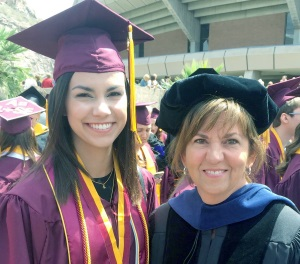The ongoing data revolution in the life sciences relies on the mathematical and computational sciences to integrate theoretical and empirical approaches and transform biology into a quantitative culture. As faculty, we must move beyond teaching mathematical skills and also entice students to transfer those skills into their particular discipline. Our approach is to develop courses that directly incorporate quantitative methods into biological content and to engage students in interdisciplinary research, exposing the connections among fields as early as possible in training.

Undergraduate Teaching and Training: Our overall goal is to balance mathematical, computational, and informatics content with the development of reasoning skills and intuition. For example, courses include projects that provide a platform for relating concepts to potential applications. Incorporating technology and active learning techniques into these projects and other assignments builds mathematical intuition by improving data analysis and visualization skills, promotes retention of course content, and exposes students to the use of technology as a problem-solving tool.

Graduate Teaching and Training: We are actively involved in multiple graduate degree programs, training students from Applied Mathematics, Bioengineering, Computer Science and Interdisciplinary Neuroscience. In every setting, we strive to educate students in approaches to solving complex biological problems, with an emphasis on building quantitative, analytical and computational skill which encouraging creativity and independence. If you are interested in working with our group as a graduate student, you will need to apply to one of these graduate programs at ASU. You can contact us for more information.

#### Introduction to Computational Molecular Biology

Due to the large volume of data generated by genome sequencing and cellular measurements of gene expression changes, computer science and mathematics have profoundly changed the science of modern biology. Computational and mathematical methods are now critical to the development of both experimental and analytical tools in genomics. This course provides students from all disciplines with an introduction to some of the basic mathematical and computational tools that have been developed to analyze, model and understand these biological data. The mathematical topics consist of discrete mathematics and probability; however, the emphasis of the course is on algorithmic techniques applied to problems motivated by molecular biology and genetics. In particular, the course focuses on sequence alignment algorithms, hidden Markov models for gene finding, and computational approaches for phylogenetic reconstruction and determining gene function. Computer labs are incorporated to implement some of the main algorithms and introduce a variety of commonly available software packages for problem solving. For example, NCBI databases, tools such as BLAST, and software packages for tree reconstruction are covered.

This course is designed to be accessible to undergraduate students from a variety of backgrounds and does not require any specific knowledge of mathematics, computer science, or molecular biology.

#### Neuroscience Data Analysis

Science is being revolutionized by datasets of unprecedented size, scope, and availability. This course will teach students how to think about data (communicating important statistical and informatics concepts) and how to work with it (using and composing computer programs to process that data into facts and figures). In this course, students apply techniques from math, engineering, art, and computer science to analyze neuroscience data and make conclusions about what the data are telling us. While this course focuses on neuro-specific examples, the tools and techniques learned in this course are general enough to be applied to any scientific discipline.

This course is designed to be accessible to undergraduate students from a variety of backgrounds and does not require any specific prior knowledge of neuroscience, statistics, or computer science.

#### Mathematical Cell Physiology

Historically, mathematics has played a huge role in the study of the mechanisms underlying cellular physiology. This course provides an introduction to the development and analysis of mathematical models for various aspects of cellular physiology including diffusion, membrane transport, ion channel kinetics, excitable membranes, and calcium dynamics. We use computational methods to perform numerical simulations and qualitative analysis for all of the models discussed in class. The course is organized around lectures (and accompanying homework assignments and computer laboratories), discussions motivated by relevant published articles, and student projects.

This course is designed for graduate students and upper level undergraduate students who have completed undergraduate differential equations course work.

#### Mathematical Neuroscience

This course covers the basic principles of modeing neurons, focusing on the electrochemical processes underlying active membranes. Topics covered include the Hodgkin-Huxley formalism for conductance based modeling, the cable equation for modeling dendritic and axonal processes, mechanisms underlying bursting, synaptic models and models of neuronal networks. Mathematical approaches include phase plane analysis, bifurcation analysis, and the use of simulation software. Student projects allow for the study of models of specific neural systems, particularly various sensory systems.

This course is designed for graduate students and upper level undergraduate students who have completed undergraduate ordinary and partial differential equations course work.

#### Mathematical Modeling

This course provides a unique opportunity for integrating different mathematical areas and for exploring the use of mathematics in applications. Students use innovative problem-solving techniques and appropriate mathematical techniques and technology to develop, analyze and simulate models for various physical and biological systems. The course also requires the use of thoughtful writing and oral communication. The mathematical techniques may include topics from discrete mathematics, ordinary and partial differential equations, dynamical systems, topology, numerical analysis, probability, and/or statistics, depending on the type of models needed to explore questions of interest. The course is organized around brief lectures, active learning activies in a computer classroom, discussions, projects, and presentations.

This course is designed for upper level undergraduate students who have completed undergraduate ordinary differential equations and linear algebra course work.